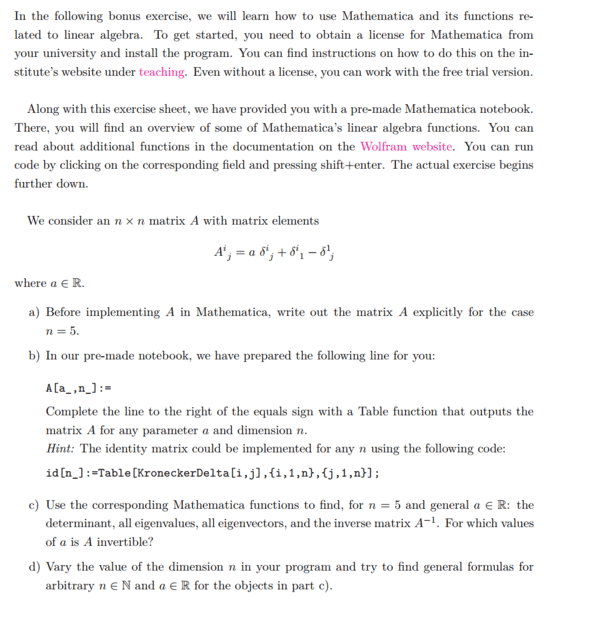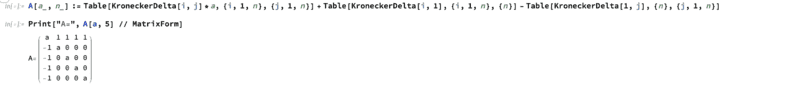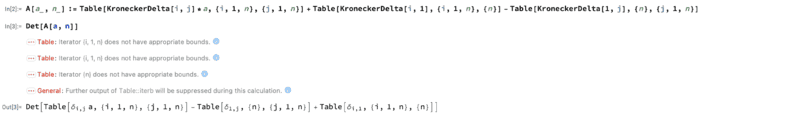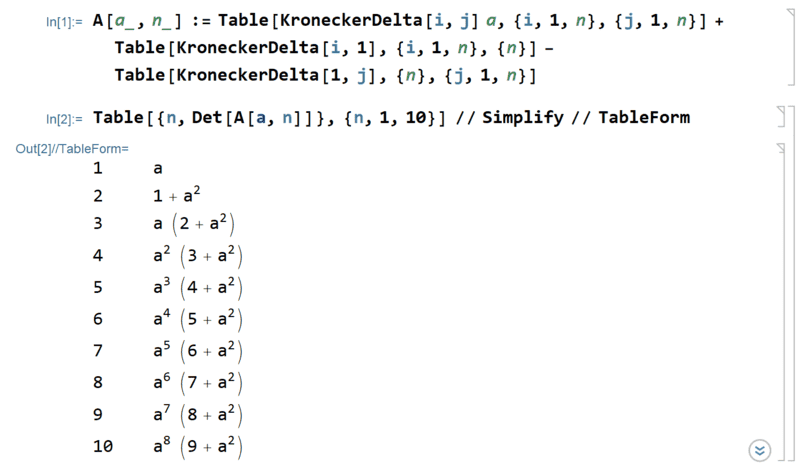# Matrices in Mathematica -- How to calculate eigenvalues, eigenvectors, determinants and inverses?

• Mathematica
• Lambda96

#### Lambda96

TL;DR Summary
How to calculate eigenvalues, eigenvectors, determinants and inverses of a general nxn matrix
Hi,

In my linear algebra homework, there is a bonus assignment where we are supposed to use Mathematica to calculate matrices and their determinants etc. here is the assignment.Unfortunately, I am a complete newbie when it comes to Mathematica, this is the first time I have worked with Mathematica.

I was able to calculate the task a to c. Here is the solution for task b and the Matrix for the case ##n=5##.I have now problems with the task d, where I should calculate the determinants, eigenvalues, etc for the general case n or rather give a formula.

For the determinant as an example I proceeded as follows and unfortunately I get the following error:Unfortunately I don't know how to fix the error or is my initial equation already wrong?

You can't leave n undefined. Mathematica can't find the rule for arbitrary n by itself. You have to calculate the result for explicit values of n, and then find by yourself the general rule.

•Lambda96 and renormalize
As DrClaude says, ##n## must be specified explicitly:The pattern is pretty obvious.

•DrClaude and Lambda96
Thanks DrClaude and renormalize for your helpThanks also renormalize for the trick with plotting n objects in Mathematica, so I could directly plot the eigenvalues from ##n=1## to ##n=10## without repeating the calculation 10 timesI could now recognize corresponding patterns for the other values•renormalize and DrClaude